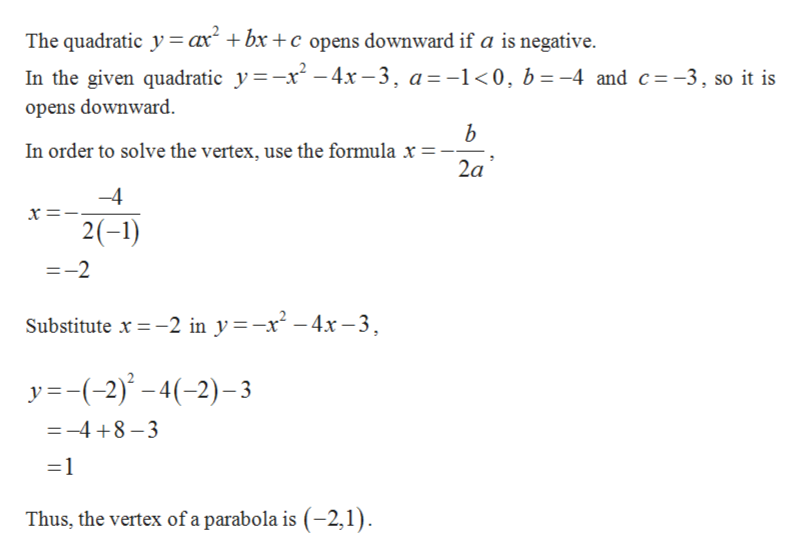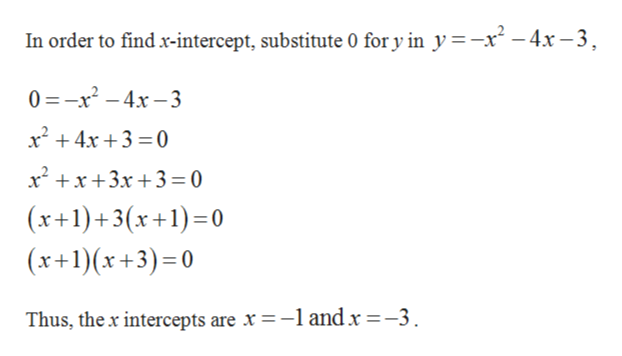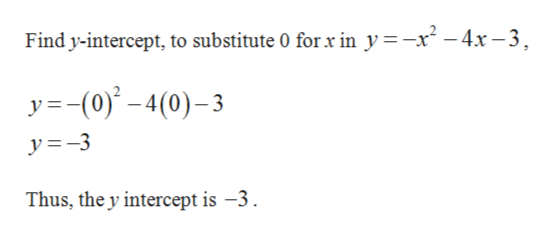# Find the vertex, y-intercept, and x intercepts of the quadratic, and then graph it.  y = -x2-4x-3

Question
80 views

Find the vertex, y-intercept, and x intercepts of the quadratic, and then graph it.

y = -x2-4x-3

check_circle

Step 1

Find the vertex of the quadratic.help_outlineImage TranscriptioncloseThe quadratic y ax +bx +c opens downward if a is negative. In the given quadratic y-x-4x-3 a=-1<0, b-4 and c -3, so it is opens downward b In order to solve the vertex, use the formula x = - 2a -4 2(-1) =-2 Substitute x-2 in y-x-4x-3 y(2)4(-2)-3 =-4 8-3 1 Thus, the vertex of a parabola is (-2,1) fullscreen
Step 2

Find x-intercept.help_outlineImage TranscriptioncloseIn order to find x-intercept, substitute 0 for y in y =-x2-4x-3 0 -x2-4x-3 x24x3 0 x x+3x + 3 0 (x+1)+3(x+1)0 (x+1x+3) 0 Thus, the x intercepts are x =-1 and x =-3 fullscreen
Step 3

Obtain y interce...help_outlineImage TranscriptioncloseFind y-intercept, to substitute 0 for x in y =-x2-4x-3 y=(0)-4(0)-3 y=3 Thus, the y intercept is -3 fullscreen

### Want to see the full answer?

See Solution

#### Want to see this answer and more?

Solutions are written by subject experts who are available 24/7. Questions are typically answered within 1 hour.*

See Solution
*Response times may vary by subject and question.
Tagged in
MathAlgebra

### Other